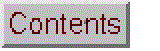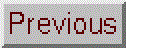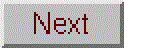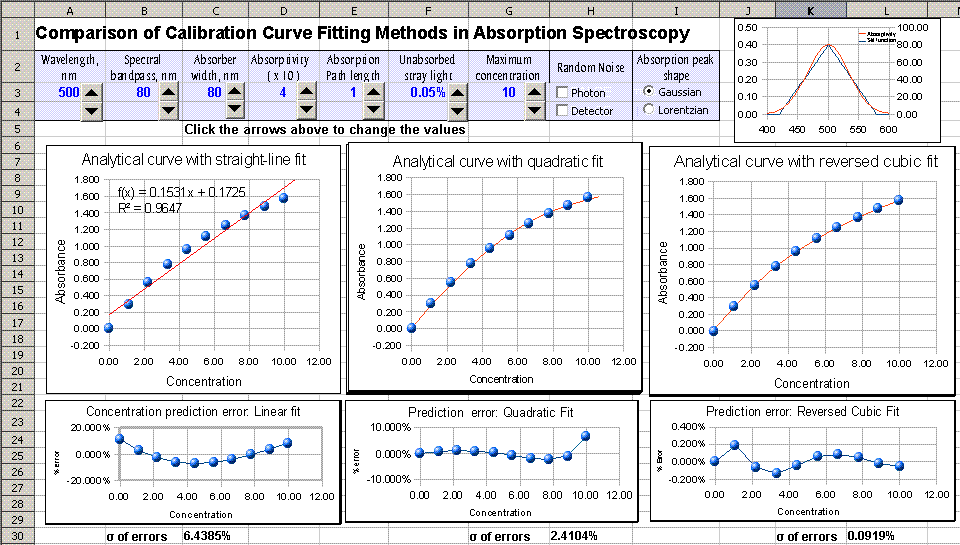# Comparison of Calibration Curve Fitting Methods in Absorption Spectroscopy

### OpenOffice Calc Version (December 31, 2013)Available in xls and ods formats.  Click to see larger graphic

The purpose of this simulation is to compare three methods of least-squares curve fitting to absorption spectrophotometry calibration curves with varying degrees of non-linearity due to polychromaticity and unabsorbed stray light (see Background information, below). The operation is similar to "Instrumental Deviation from Beer's Law"; it computes the measured absorbance and plots the analytical curve (absorbance vs concentration) for a simulated series of absorber concentrations measured in a simulated absorption spectrophotometer with variable spectral bandpass and unabsorbed stray light. You control the maximum absorptivity, path length, half-width of the absorber, the slit width of the monochromator, and the percent stray light. (Number wheels below each of these parameters allow you to change the values quickly without typing. The analytical curves change dynamically as the variables are changed). The calibration curve is fit using three different least-squares methods, shown from left to right:
1. A first-order (straight line) fit of measured absorbance A (y-axis) vs concentration C (x-axis). The model equation is A = slope * C + intercept. The is the most common and straightforward method, but it obviously can not compensate for non-linearity. This fit is performed using the SLOPE and INTERCEPT functions (on Sheet2 of the spreadsheet, in cells G51, G52, and G53). The concentration of unknown samples is given by (A - intercept) / slope where A is the measured absorbance and slope and intercept from the first-order fit. If you would like to use this method of calibration for your own data, download a fill-in-the-blanks worksheet, in Excel or OpenOffice Calc format.
2. A quadratic fit of measured absorbance A (y-axis) vs concentration C (x-axis). The model equation is A = a*C2 + b*C + c. This fit is performed using the LINEST function (on Sheet2 in cells E57-G57). The concentration of unknown samples is calculated by solving this equation for C using the classical "quadratic formula", namely C = (-b+SQRT(b2-4*a*(c-A)))/(2*a), in cells E58:N58, where A = measured absorbance, and a, b, and c are the three coefficients from the quadratic fit.  If you would like to use this method of calibration for your own data, download in Excel or OpenOffice Calc format.
3. A reversed cubic fit of concentration C (y-axis) vs measured absorbance A (x-axis). The model equation is C = a*A3 + b*A2 + c*A + d.  This method reverses the usual order of axes in order to avoid the need to solve a cubic equation when the calibration equation is solved for C and used to convert the measured signals of the unknowns into concentration.  (This sort of short-cut is commonly done in least-squares curve fitting, at least by non-statisticians, to avoid mathematical messiness). This fit is performed using the LINEST function (on Sheet2 in cells E73-H73). The concentration of unknown samples is calculated directly by a*A3+b*A2+c*A+d, where A is the measured absorbance, and a, b, c, and d are the four coefficients from the cubic fit. If you would like to use this method of calibration for your own data, download in Excel or OpenOffice Calc format.
Below each calibration curve is a plot of the concentration prediction error, the percent difference between the actual concentration of each standard in the simulation and the concentration predicted from its measured absorbance according to the curve fit equation, expressed as a percentage of the highest standard concentration.  For ease in comparison, the standard deviation of all the concentration prediction errors is computed and displayed to the left as the "σ of errors". Version 2 allows the user to select the quantity to plot vs concentration: either absorbance (log(Io/I)) or absorption, showing that it is better to compute absorbance rather than absorption, even if you use a cubic least-squares fit to the calibration curve.

Assumptions of this simulation: The true monochromatic absorbance follows the Beer-Lambert Law; the absorber has a single Gaussian or Lorentzian absorption band (selectable by user); the spectral width of the light source is much greater than the monochromator spectral bandpass; the monochromator has a triangular slit function (i.e. entrance and exit slits are equal); the absorption path length and absorber concentration are both uniform across the light beam; the spectral response of the detector is much wider that the spectral bandpass of the monochromator. The current versions include the optional addition of photon and detector noise to both the incident and transmitted beam intensities; it is assumed that both beams are subject to random and uncorrelated noise.

View Equations (.pdf)
Download Version 2 (includes selection of absorbance or transmission) : in OpenOffice format (.ods)
; in Excel format (.xls)

Worksheets for linear and non-linear calibration curves for your own data
Instrumental Deviation from Beer's Law
Signal-to-noise ratio of absorption spectrophotometry
Effect of Slit Width on Signal-to-Noise Ratio in Absorption Spectroscopy
Error propagation in Analytical Calibration
The Transmission Fitting (TFit) Method applies curve fitting to the spectra rather than the calibration data.
Curve fitting multiple overlapping peaks
Interactive Peak Fitter

Background

In absorption spectroscopy, the intensity I of light passing through an absorbing sample is given by the Beer-Lambert Law:

I = Io*10-(alpha*L*c)

where “Io” is the intensity of the light incident on the sample, “alpha” is the absorption coefficient of the absorber, “L” is the distance that the light travels through the material (the path length), and “c” is the concentration of absorber in the sample. The variables I, Io, and alpha are all functions of wavelength; L and c are scalar. In conventional applications, measured values of I and Io are used to compute the absorbance, defined as

A = log(Io/I)
Ideally, absorbance defined in this way is proportional to concentration, which simplifies analytical calibration. A plot of A vs C is called the analytical curve or the calibration curve.

It's important to understand that the "deviations" from the Beer-Lambert Law discussed here are not actually failures of this law but rather apparent deviations caused by failures of the measuring instrument to adhere to the conditions under which the law is derived. The fundamental requirement under which then Beer-Lambert Law is derived is that every photon of light striking the detector must have an equal chance of absorption. Thus, every photon must have the same absorption coefficient alpha, must pass through the same absorption path length, L, and must experience the same absorber concentration, c. Anything that violates these conditions will lead to an apparent deviation from the law.

For example, any real spectrometer has a finite spectral resolution, meaning that the intensity reading at one wavelength setting is actually an average over a small spectral interval. Specifically, what is actually measured is a convolution of the true spectrum of the absorber and the instrument function (or "slit function"). If the absorption coefficient alpha varies over that interval, then the calculated absorbance will no longer be linearly proportional to concentration. This is called the “polychromicity” error and it results in a gradual concave-down curvature of the analytical curve.

Another source of instrumental non-ideality is stray light, which is any light striking the detector whose wavelength is outside the spectral bandpass of the monochromator or which has not passed through the sample.  Since in most cases the wavelength setting of the monochromator is the peak absorption wavelength of the analyte, it therefore follows that any light outside this spectral range is less absorbed. The most serious effect is caused by stray light that is not absorbed by the analyte at all; this is called unabsorbed stray light. This effect also leads to a concave-down curvature of the analytical curve, but the effect is relatively minor at low absorbances and increases quickly at high absorbances. Ultimately, unabsorbed stray light results in a flat plateau in the analytical curve at an absorbance of -log(fsl), where fsl is the fractional stray light.

There are two other potential sources of deviation that are actually not so serious in laboratory applications of absorption spectrophotometry, because they are relatively easily avoided: those are unequal light path lengths and unequal absorber concentration across the light beam.  In most laboratory applications, the samples are measured in square cuvettes to insure a constant path length for all photons.  (When round test-tube sample cells are used, the light beam passing through the sample is restricted to the central region of the sample tube in order to minimize this effect).  Solution samples are carefully mixed before measurement to insure homogeneity.

The simulation here includes the polychromaticity and unabsorbed stray light errors (but not the path length and sample inhomogeneity errors, because they are seldom important in laboratory practice). The simulation operates like any numerical integration, by slicing up the spectral range viewed by the detector into a large number of small slices and assuming that the Beer-Lambert Law applies over each small slice separately. The sample absorption is represented in this simulation by a single absorption band of either Gaussian or Lorentzian shape (selectable by the user) and adjustable width.  The spectral bandpass of the monochromator is represented by a triangular function of adjustable width.  Then all the separate slices are summed up to represent the incident and transmitted light signal measured by the detector.  As it turns out, one does not need to use very many slices to obtain a good model of the operation of a typical absorption spectrophotometric measurement.

Student handout. Note: Instructors are encouraged to copy, paste, and edit this material as needed to customize for their own terminology and instructional aims.

## Comparison of Calibration Curve Fitting Methods in Absorption Spectroscopy

Open either the Excel xls or the Calc ods version of BeersLawCurveFit. This spreadsheet simulates a visible absorption spectroscopy measurement, including the instrumental deviations from the Beer-Lambert Law (a.k.a. Beer's Law) caused by polychromatic light and unabsorbed stray lightThis spreadsheet is similar to Instrumental Deviation from Beer's Law; the controls are similar to that simulation; the main difference is that, instead of just a linear least-squares fit to the calibration curve, this simulation compares the linear fit with two non-linear (so-called "curvelinear") fitting methods, the "quadratic" and the "reversed cubic" fits.

In the
quadratic method, the measured absorbance A (y-axis) vs concentration C (x-axis) is fit to a quadratic model, A = aC2 + bC + c, using standard least-squares techniques, yielding the three coefficients a, b, and cThereafter, the concentration of unknown samples is calculated by solving this equation for C using the classical "quadratic formula" using those same coefficients, namely C = (-b2+SQRT(b-4*a*(c-A)))/(2*a), where A = measured absorbance, and a, b, and c are the three coefficients from the quadratic fit.

In the "reversed cubic" fit, the measured absorbances of the standard solutions are treated as the independent variable and the concentrations of the standard solutions are treated as the dependent variable. Then the calibration data set is fitted to a cubic equation, C = aA3 + bA2 + cA + d, where C is concentration, A is absorbance, and a, b, c, and d are the coefficients from the least-squares fit. Thereafter, the concentrations of unknown samples are calculated from their measured absorbances by using those same coefficients:  C = aA3 + bA2 + cA + d.  Clearly, both curvilinear methods are more complicated computationally than a simple straight-line fit, but they can handle curvature in the calibration curve. The cubic version is capable of handling more complex curve shapes.

The purpose of this simulation is to discover which of these methods is best able to fit the kind of non-linearity that results from the polychromatic and stray light errors in absorption spectroscopy.  Moreover, the simulation can demonstrate how the use of a curvilinear fitting methods can influence the optimum choice of slit width and spectral bandpass.

1. Start the experiment with a
nearly ideal case. Set wavelength = 500 nm, spectral bandpass = 10 nm, absorber width = 200 nm, maximum absorptivity = 4, path length = 1 cm, unabsorbed stray light = 0; maximum concentration = 10, no photon or detector noise (both boxes unchecked) and Gaussian peak shape.  You can see that the ideal absorbances (red line), the measured absorbances (blue dots), and the least-squares fit (blue line) are essentially identical, even at the highest concentrations (where the absorbance is 4), showing that the instrument readings follow Beer's Law.  The concentration prediction error plots below each calibration curve show almost no error due to curve-fitting and the "σ of errors" is zero in all cases.  In this case the linear curve-fit methods works perfectly, so you really don't need to use more complicated methods.

2.
Unabsorbed stray light limit only. Leave the settings as they were, except increase the unabsorbed stray light to 0.1%. Now you can see some serious non-linearity at high absorbances, caused by the stray light, as the absorbance approaches the plateau at 3.0 absorbance. Right away, you can see that the straight-line fit fails badly, and that the quadratic and cubic fits are a little better but not perfect. The "σ of errors" number at the bottom provides a quick single-number comparison: the curvilinear methods do a little better than the straight-line fit, but even the cubic fit does not do a very good job if fitting the curvature in this case.

3. Effect of spectral bandpass.
Leave the settings as they were in #2, except reduce the absorber width to 100 nm. Then increase the spectral bandpass step-by-step and watch what happens.  You can see the slope of the analytical curve decreases slightly as spectral bandpass increases. Why? This is caused by the polychromatic light effect, as the increasingly wide spectral bandpass includes wavelengths where the analyte's absorptivity is lower.  But the reduced slope also means that the absorbances at high concentrations are lower, and therefore stray light effect is less serious. You can also see that the shape of the calibration curve changes as the spectral bandpass increases, exhibiting a more gradual concave-down curvature. As the result of this, the quadratic and cubic fits are better able to model the curvature, and the σ of errors actually decreases as the spectral bandpass increases, even though the increasingly serious polychromatic effect itself is expected to increase non-linearity. At a spectral bandpass of 90 nm, the σ of errors drops to 1.1 for the quadratic fit and only 0.08% for the cubic fit! So the bottom line is that you should not automatically assume that the smallest slit width will always give you the best calibration linearity or the smallest calibration errors, at least when stray light is a factor.

4. Effect of random noise. But there is another reason why the best results in absorption spectroscopy are not always obtained at the smallest slit width: noise. This aspect is explored in more detail in the simulations "Effect of Slit Width on Signal-to-Noise Ratio in Absorption Spectroscopy" and "
Signal-to-noise ratio of absorption spectrophotometry". The present simulation includes two of the most commonly-encountered types of noise: photon noise and detector noise. Photon noise (often the limiting noise in instruments that use photomultiplier detectors) is proportional to the square root of light intensity. Detector noise (often the limiting noise in instruments that use solid-state array detectors such as CCD and CID detectors) is independent of the light intensity. These sources of noise can be introduced into the simulation by clicking the check boxes under "Random Noise". When either of these types of noise is included in the simulation, these noises will be added to the incident and transmitted intensities (Izero and I, respectively) from which the absorbances are computed, resulting in some random variation in the measured absorbances within one calibration curve and between recalculations of the spreadsheet (press f9 to recalculate).  The relative effect of the noise depends strongly on the slit width and the spectral bandpass: at small slit widths, the values of Izero and I are both low, resulting in poor signal-to-noise ratio and a high random variation in measured absorbance.  As the slit width increases, the intensities increase and the signal-to-noise ratio improves, resulting in less  random variation in measured absorbance, but also the slope of the analytical curve decreases, which reduces the effect of stray light but increases the effect of polychromatic light.  The net effect is that there is an optimum range of slit widths that is the best trade-off between poor signal-to-noise ratio at the low end and unacceptable non-linearity at the high end.  This optimum slit width usually corresponds to a spectral bandpass roughly equal to the width of the absorption peak, depending upon the type of noise and the curve fitting method used.

The effect of noise can be dramatically demonstrated by setting
wavelength = 500 nm, spectral bandpass = 10 nm, absorber width = 80 nm, maximum absorptivity = 4, path length = 1 cm, unabsorbed stray light = 0.1%; maximum concentration = 10, and checking the boxes for both photon and detector noise.  The calibration curve now shows a substantial degree of random noise in the absorbances, especially at high absorbance where the transmitted intensity (I) is  therefore the signal-to-noise ratio is very low.  The "σ of errors" number is high for all three curve fitting methods.  Now, increase the spectral bandpass and watch what happens.  The random noise gradually decreases (because the intensities I and Izero are both increased) and the concentration prediction errors begins to drop.  Eventually as the spectral bandpass approaches the absorber width, the calibration curve becomes smoothly non-linear, but its shape is easily modeled by the cubic curve fit. The result is that the concentration prediction error for the straight-line fit starts to go back up at high spectral bandpass, but for the cubic fit, it continues to drop down to near 0.1%.

Conclusion: The most common sources of deviation from Beer's Law, stray light and polychromatic radiation, lead to concave-down non-linearity in the calibration curves, which can be fit approximately (but not exactly) using quadratic or cubic least-squares fits.  In most cases, the cubic fit is better that the quadratic.  In the case of an extreme unabsorbed stray light plateau, neither method works well.

The theoretical requirement for adherence to the Beer-Lambert Law is that the incident light be monochromatic, which implies that the smallest possible slit width and spectral bandpass be used. Nevertheless, this simulation shows that, in the presence of stray light and random photon or detector noise, a larger
slit width and spectral bandpass will give better signal-to-noise ratio and concentration prediction error, especially if a curvilinear least-squares method (such as the reversed cubic method) is used to fit the calibration data.

Note: The non-linearity observed here has its origin in the spectral domain (intensity vs wavelength)
, not in the calibration domain (absorbance vs concentration). Therefore it should be no surprise that curve fitting in the calibration domain is not a perfect solution. An alternative and more advanced approach to quantitative measurement in absorption spectrophotometry is to apply curve fitting to the spectral domain where the problem originates; this is what the "Transmission fitting" method is all about.

(c) 1991, 2015. This page is part of Interactive Computer Models for Analytical Chemistry Instruction, created and maintained by Prof. Tom O'Haver , Professor Emeritus, The University of Maryland at College Park. Comments, suggestions and questions should be directed to Prof. O'Haver at toh@umd.edu.
Number of unique visits since May 17, 2008: